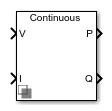# Power Measurement (Three-Phase)

Calculate three-phase real and reactive power

•Libraries:
Simscape / Electrical / Control / Measurements

## Description

The Power Measurement (Three-Phase) block measures the real and reactive power of an element in a three-phase network. The block outputs the power quantities for each frequency component you specify in the selected symmetrical sequence.

Use this block to measure power for both sinusoidal and nonsinusoidal periodic signals. For single-phase power measurement, consider using the Power Measurement block.

Set the Sample time parameter to `0` for continuous-time operation, or explicitly for discrete-time operation.

Specify a vector of all frequency components to include in the power output using the Harmonic numbers parameter:

• To output the DC component, specify `0`.

• To output the component corresponding to the fundamental frequency, specify `1`.

• To output components corresponding to higher-order harmonics, specify `n > 1`.

### Equations

For each specified harmonic k, the block calculates the real power Pk and reactive power Qk for the specified sequence from the phasor equation:

`${P}_{k}+j{Q}_{k}=\frac{3}{2}\left({V}_{k}{e}^{j{\theta }_{{V}_{k}}}\right)\left(\overline{{I}_{k}{e}^{j{\theta }_{{I}_{k}}}}\right),$`

where:

• ${V}_{k}{e}^{j{\theta }_{{V}_{k}}}$ is the phasor representing the k-component voltage of the selected sequence.

• $\overline{{I}_{k}{e}^{j{\theta }_{{I}_{k}}}}$ is the complex conjugate of ${I}_{k}{e}^{j{\theta }_{{I}_{k}}}$, the phasor representing the k-component current of the selected sequence.

Select the symmetrical sequence used in the power calculation using the Sequence parameter:

• `Positive`:

`${V}_{k}{e}^{j{\theta }_{{V}_{k}}}={V}_{k+}{e}^{j{\theta }_{{V}_{k+}}},\text{\hspace{0.17em}}{I}_{k}{e}^{j{\theta }_{{I}_{k}}}={I}_{k+}{e}^{j{\theta }_{{I}_{k+}}}$`

• `Negative`:

`${V}_{k}{e}^{j{\theta }_{{V}_{k}}}={V}_{k-}{e}^{j{\theta }_{{V}_{k-}}},\text{\hspace{0.17em}}{I}_{k}{e}^{j{\theta }_{{I}_{k}}}={I}_{k-}{e}^{j{\theta }_{{I}_{k-}}}$`

• `Zero`:

`${V}_{k}{e}^{j{\theta }_{{V}_{k}}}={V}_{k0}{e}^{j{\theta }_{{V}_{k0}}},\text{\hspace{0.17em}}{I}_{k}{e}^{j{\theta }_{{I}_{k}}}={I}_{k0}{e}^{j{\theta }_{{I}_{k0}}}$`

The block calculates the symmetrical set of +-0 voltage phasors from the set of abc voltage phasors using the symmetrical components transform S:

`$\left[\begin{array}{c}{V}_{k+}{e}^{j{\theta }_{{V}_{k+}}}\\ {V}_{k-}{e}^{j{\theta }_{{V}_{k-}}}\\ {V}_{k0}{e}^{j{\theta }_{{V}_{k0}}}\end{array}\right]=S\left[\begin{array}{c}{V}_{ka}{e}^{j{\theta }_{{V}_{ka}}}\\ {V}_{kb}{e}^{j{\theta }_{{V}_{kb}}}\\ {V}_{kc}{e}^{j{\theta }_{{V}_{kc}}}\end{array}\right].$`

The block obtains this set of abc voltage phasors from the three-phase input voltage V(t) as:

`$\left[\begin{array}{c}{V}_{ka}{e}^{j{\theta }_{{V}_{ka}}}\\ {V}_{kb}{e}^{j{\theta }_{{V}_{kb}}}\\ {V}_{kc}{e}^{j{\theta }_{{V}_{kc}}}\end{array}\right]=\frac{2}{T}{\int }_{t-T}^{t}V\left(t\right)\mathrm{sin}\left(2\pi kFt\right)dt+j\frac{2}{T}{\int }_{t-T}^{t}V\left(t\right)\mathrm{cos}\left(2\pi kFt\right)dt,$`

where T is the period of the input signal, or equivalently the inverse of its base frequency F.

The block calculates the symmetrical set of current phasors in the same way as it does the voltage.

If the input signals have a finite number of harmonics n, the total real power P and total reactive power Q for the specified sequence can be calculated from their components:

`$P=\sum _{k=0}^{n}{P}_{k}$`

`$Q=\sum _{k=1}^{n}{Q}_{k}.$`

The summation for Q does not include the DC component (k = 0), because this component only contributes to real power.

## Ports

### Input

expand all

Three-phase voltage across element from which to measure power, in V.

Data Types: `single` | `double`

Three-phase current through element from which to measure power, in A.

Data Types: `single` | `double`

### Output

expand all

Real power for selected frequency components, in W. If the Harmonic numbers parameter value is a scalar, this output is also a scalar.

Data Types: `single` | `double`

Reactive power for selected frequency components, in var. If the Harmonic numbers parameter value is a scalar, this output is also a scalar.

Data Types: `single` | `double`

## Parameters

expand all

Fundamental frequency corresponding to component k=1.

Frequency components to include in the output. Specify either a scalar value corresponding to the desired component or a vector of all desired components.

• The value k = 0 corresponds to the DC component.

• The value k = 1 corresponds to the fundamental frequency.

• Values k > 1 correspond to higher-level harmonics.

If you specify a vector, the order of the power outputs correspond to the order of this vector.

Symmetrical sequence of the power output.

Time between consecutive block executions. During execution, the block produces outputs and, if appropriate, updates its internal state. For more information, see What Is Sample Time? and Specify Sample Time.

For continuous operation, set this property to `0`. For discrete operation, specify the sample time explicitly as a positive number. This block does not support inherited sample time.

If this block is in a masked subsystem, or other variant subsystem that allows either continuous and discrete operation, promote the sample time parameter. Promoting the sample time parameter ensures correct switching between the continuous and discrete implementations of the block. For more information, see Promote Block Parameters on a Mask.

## Version History

Introduced in R2017b